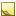﻿ RGB Structure# RGB Structure

RGB components.

Namespace:  Accord.Imaging
Assembly:  Accord.Imaging (in Accord.Imaging.dll) Version: 3.8.0Syntax
```[SerializableAttribute]
public struct RGB```

The RGB type exposes the following members.Constructors
NameDescriptionRGB(Color)
Initializes a new instance of the RGB class.RGB(Byte, Byte, Byte)
Initializes a new instance of the RGB class.RGB(Byte, Byte, Byte, Byte)
Initializes a new instance of the RGB class.
TopProperties
NameDescriptionColor
Color value of the class.
TopMethods
NameDescriptionEquals
Indicates whether this instance and a specified object are equal.
(Inherited from ValueType.)GetHashCode
Returns the hash code for this instance.
(Inherited from ValueType.)GetType
Gets the Type of the current instance.
(Inherited from Object.)ToString
Returns the fully qualified type name of this instance.
(Inherited from ValueType.)
TopOperators
NameDescription(RGB to HSL)
Performs an explicit conversion from RGB to HSL.(RGB to YCbCr)
Performs an explicit conversion from RGB to YCbCr.
TopFields
NameDescriptionA
Index of alpha component for ARGB images.Alpha
Alpha component.B
Index of blue component.Blue
Blue component.G
Index of green component.Green
Green component.R
Index of red component.Red
Red component.
TopExtension Methods
NameDescriptionHasMethod
Checks whether an object implements a method with the given name.
(Defined by ExtensionMethods.)IsEqual
Compares two objects for equality, performing an elementwise comparison if the elements are vectors or matrices.
(Defined by Matrix.)To(Type)Overloaded.
Converts an object into another type, irrespective of whether the conversion can be done at compile time or not. This can be used to convert generic types to numeric types during runtime.
(Defined by ExtensionMethods.)ToTOverloaded.
Converts an object into another type, irrespective of whether the conversion can be done at compile time or not. This can be used to convert generic types to numeric types during runtime.
(Defined by ExtensionMethods.)
TopRemarks

The class encapsulates RGB color components and can be used to implement logic for reading, writing and converting to and from RGB color representations.Note
The PixelFormat.Format24bppRgb actually refers to a BGR pixel format.Examples

The following examples show how to convert to and from various pixel representations:

```// This example shows how to convert to and from various
// pixel representations. For example, let's say we start
// with a RGB pixel with the value (24, 250, 153):
RGB rgb = new RGB(red: 24, green: 250, blue: 153);

// The value of this pixel in HSL format would be:
HSL hsl = HSL.FromRGB(rgb);       // should be (154, 0.9576271, 0.5372549)

// The value of this pixel in YCbCr format would be:
YCbCr yCbCr = YCbCr.FromRGB(rgb); // should be (0.671929836, -0.0406815559, -0.412097245)

// Note: we can also convert using casting:
HSL a = (HSL)rgb;
YCbCr b = (YCbCr)rgb;```
```// This example shows how to convert to and from various
// pixel representations. For example, let's say we start
// with a HSL pixel with the value (123, 0.4, 0.8):
HSL hsl = new HSL(hue: 123, saturation: 0.4f, luminance: 0.8f);

// The value of this pixel in RGB format would be:
RGB rgb = hsl.ToRGB();       // should be (183, 224, 185)

// The value of this pixel in YCbCr format would be:
YCbCr yCbCr = YCbCr.FromRGB(rgb); // should be (0.8128612, -0.0493462719, -0.0679121539)

// Note: we can also convert using casting:
RGB a = (RGB)hsl;
YCbCr b = (YCbCr)hsl;```
```// This example shows how to convert to and from various
// pixel representations. For example, let's say we start
// with a YCbCr pixel with the value (0.8, 0.2, 0.5):
YCbCr yCbCr = new YCbCr(y: 0.8f, cb: 0.2f, cr: 0.5f);

// The value of this pixel in RGB format would be:
RGB rgb = yCbCr.ToRGB();  // should be (255, 95, 255)

// The value of this pixel in HSL format would be:
HSL hsl = HSL.FromRGB(rgb); // should be (299, 0.99999994, 0.6862745)

// Note: we can also convert using casting:
RGB a = (RGB)yCbCr;
HSL b = (HSL)yCbCr;```See Also